# Texas Go Math Grade 2 Lesson 18.2 Answer Key Time to 5 Minutes

Refer to our Texas Go Math Grade 2 Answer Key Pdf to score good marks in the exams. Test yourself by practicing the problems from Texas Go Math Grade 2 Lesson 18.2 Answer Key Time to 5 Minutes.

## Texas Go Math Grade 2 Lesson 18.2 Answer Key Time to 5 Minutes

Essential Question
How do you tell and show time to five minutes?

Explore
Draw the hour hand and the minute hand to show the time.For The Teacher

• Read the following story and have children draw the hour and minute hands to show each time. Sofia goes to music at 10:30. She goes to the playground at 11:00. She eats lunch at 11:30. Show the times Sofia does these things.

Answer:Children drawn the hour hand and the minute hand to show each time.
Explanation:
In the above image we can observe three clock’s with different time.
Sofia goes to music at 10:30. The first clock represents the Sofia’s Music class time.
The hour hand is between 10 and 11
and the minute hand is on 6, which makes 30 minutes on the clock
Making it as 10:30 on the clock
She goes to the playground at 11:00. The second clock represents the Sofia’s playground time.
The hour hand is on 11
and the minute hand is on 12, which makes a whole hour
Making it as 11:00 on the clock
She eats lunch at 11:30. The third clock represents the Sofia’s lunch time.
The hour hand is between 11 and 12
and the minute hand is on 6, which makes 30 minutes on the clock
Making it as 11:30 on the clock

Math Talk
Mathematical Processes
Describe where the minute hand points to show half past the hour.
Half past means 30 minutes past an hour.
The number on the clock that the hour hand has just gone past tells us which hour we are half an hour past.
For example at half past one the larger minutes hand is pointing at the 6 and the smaller hour hand is halfway between the 1 and the 2.

Model and Draw
What does it mean when the minute hand points to the 7?

Count by fives until you reach the 7.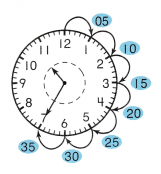The hour hand points between the 10 and the 11. The minute hand points to the 7.
The time is _____
The time is 10 : 35.
Explanation:
In the above image we can observe the hour hand points between the 10 and the 11.
The minute hand points to the 7.
If the minute hand is on 6, which makes 30 minutes on the clock , add five minutes to get to 7
Count by fives until you reach the 7.
So, the time is 10 : 35.

Digital clocks also show the time.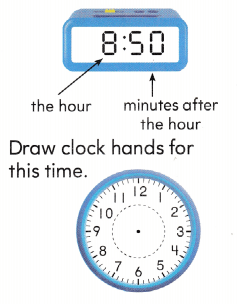Answer:Explanation:
The hour hand is between 8 and 9
and the minute hand is on 10 , which makes 50 minutes on the clock ,
for example , if the minute hand is on 6 , makes 30 minutes
but here it is on 10 that means add 20 minutes to the 30 minutes which results in 50 minutes
Making it as 8:50 on the clock

Share and Show

Look at the clock hands. Write the time.

Question 1.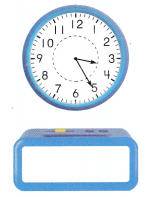Answer: The time on the clock is 3:25

Explanation:The hour hand is between 3 and 4
and the minute hand is on 5 , which makes 25 minutes on the clock ,
for example , if the minute hand is on 6 , makes 30 minutes
but here it is on 5 that means 5 minutes less to the 30 minutes which results in 25 minutes
Making it as 3:25 on the clock

Question 2.Answer: The time on the clock is 9:05

Explanation: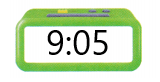The hour hand is on 9
It takes 5 minutes for the minute hand to move from one number to the next number on a clock
and the minute hand is on 1 , which makes 5 minutes on the clock ,
Making it as 9:05

Question 3.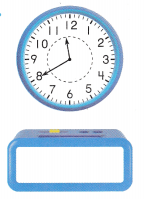Answer: The time on the clock is 11:40

Explanation: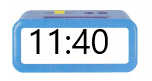The hour hand is between 11 and 12
and the minute hand is on 8 , which makes 40 minutes on the clock ,
for example , if the minute hand is on 6 , makes 30 minutes
but here it is on 8 that means add 10 minutes to the 30 minutes which results in 40 minutes
Making it as 11:40 on the clock

Question 4.Answer: The time on the clock is 12:50

Explanation: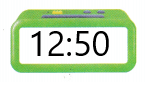The hour hand is between 12 and 1
and the minute hand is on 10 , which makes 50 minutes on the clock ,
for example , if the minute hand is on 6 , makes 30 minutes
but here it is on 10 that means add 20 minutes to the 30 minutes which results in 50 minutes
Making it as 12:50 on the clock

Question 5.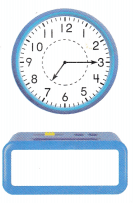Answer: The time on the clock is 7:15

Explanation:The hour hand is between 7 and 8
and the minute hand is on 3, which makes 15 minutes on the clock
That is adding up the 5 minutes three times can make a whole 15 minutes.
Making it as 7:15 on the clock

Question 6.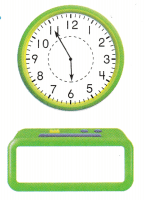Answer: The time on the clock is 5:55

Explanation: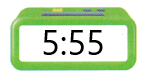The hour hand is between 5 and  close 6
and the minute hand is on 11 , which makes 55 minutes on the clock ,
Here 6o minutes makes an hour, so 55 minutes means 5 minutes less than a hour
Making it as 5:55 on the clock

Problem Solving

Look at the time. Draw the minute hand to show the same time.

Question 7.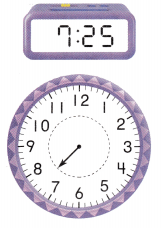Answer: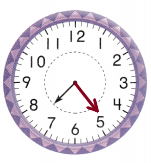Explanation:
The hour hand is between 7 and 8
and the minute hand is on 5 , which makes 25 minutes on the clock ,
for example , if the minute hand is on 6 , makes 30 minutes
but here it is on 5 that means 5 minutes less to the 30 minutes which results in 25 minutes
Making it as 7:25 on the clock

Question 8.Answer: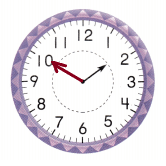Explanation:
The hour hand is between 1 and 2
and the minute hand is on 10 , which makes 50 minutes on the clock ,
for example , if the minute hand is on 6 , makes 30 minutes
but here it is on 10 that means add 20 minutes to the 30 minutes which results in 50 minutes
Making it as 1:50 on the clock

Question 9.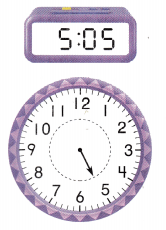Answer: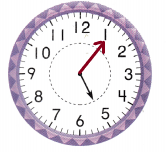Explanation:
The hour hand is on 5
and the minute hand is on 1 , which makes 5 minutes on the clock ,
Making it as 5:55 on the clock

Draw the clock hands to show the time. Then write the time.

Question 10.
Multi-Step My hour hand points between the 8 and the 9. In 35 minutes it will be the next hour. What time is it?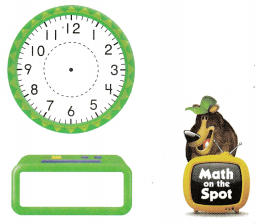Answer: The time on the clock  is 8:25

Explanation:Given, In 35 minutes it will be the next hour. that is 35 and 25 together makes a hour.
The hour hand is between 8 and 9
and the minute hand is on 5 , which makes 25 minutes on the clock ,
for example , if the minute hand is on 6 , makes 30 minutes
but here it is on 5 that means 5 minutes less to the 30 minutes which results in 25 minutes
Making it as 7:25 on the clock

Question 11.
H.O.T. Mr. Brady fixes broken computers. Look at the start and finish times for his work on one computer. How many minutes did he work on the computer?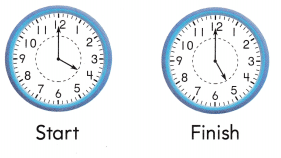__ minutes
Answer: Mr. Brady worked for 60 minutes or a whole hour on the computer.

Explanation:
The starting clock  points at 4:00
The finishing clock points at 5:00
The difference between the time is full hour or 60 minutes.
So, Mr. Brady worked for 60 minutes or a whole hour on the computer.

Question 12.
Representations Javier looks at the clock. He sees that it is time for music class. Which digital clock shows the same time as the clock to the right?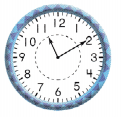Answer:Explanation:
In the given clock, The hour hand points at 11
The minute hand points at 2 , that means 10 minutes on the clock
Making it as 11:10.

Question 13.
Nora starts studying at the time shown on this digital clock. Which clock shows the same time?Answer: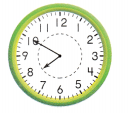Explanation:
The hour hand is between 7 and 8
and the minute hand is on 10 , which makes 50 minutes on the clock ,
for example , if the minute hand is on 6 , makes 30 minutes
but here it is on 10 that means add 20 minutes to the 30 minutes which results in 50 minutes
Making it as 7:50 on the clock

Question 14.
Texas Test Prep What time is shown on this clock?
(A) 1:45
(B) 12:45
(C) 9:05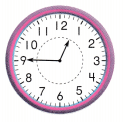Explanation:
The hour hand is between 12 and 1
and the minute hand is on 9 , which makes 45 minutes on the clock ,
for example , if the minute hand is on 6 , makes 30 minutes
but here it is on 9 that means add 15 minutes to the 30 minutes which results in 45 minutes
Making it as 12:45 on the clock

Take Home Activity

• Have your child draw a large clock face and use two pencils as clock hands to show some different times.

### Texas Go Math Grade 2 Lesson 18.2 Homework and Practice Answer Key

Look at the clock hands. Write the time.

Question 1.Answer: The time on the clock is 8:35

Explanation: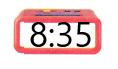The hour hand is between 8 and 9
and the minute hand is on 7 , which makes 35 minutes on the clock ,
for example , if the minute hand is on 6 , makes 30 minutes
but here it is on 7 that means add 5 minutes to the 30 minutes which results in 35 minutes
Making it as 8:35 on the clock

Question 2.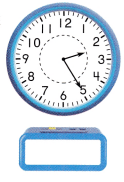Answer: The time on the clock is 2:25

Explanation:The hour hand is between 2 and 3
and the minute hand is on 5 , which makes 25 minutes on the clock ,
for example , if the minute hand is on 6 , makes 30 minutes
but here it is on 5 that means 5 minutes less to the 30 minutes which results in 25 minutes
Making it as 2:25 on the clock

Question 3.Answer: The time on the clock is 7:50

Explanation: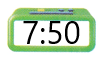The hour hand is between 7 and 8
and the minute hand is on 10 , which makes 50 minutes on the clock ,
for example , if the minute hand is on 6 , makes 30 minutes
but here it is on 10 that means add 20 minutes to the 30 minutes which results in 50 minutes
Making it as 7:50 on the clock

Question 4.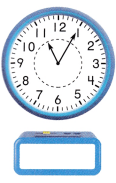Answer: The time on the clock is 11:05

Explanation: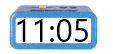The hour hand is on 11
It takes 5 minutes for the minute hand to move from one number to the next number on a clock
and the minute hand is on 1 , which makes 5 minutes on the clock ,
Making it as 11:05

Question 5.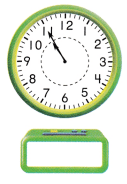Answer: The time on the clock is 10:55

Explanation:The hour hand is between 10 and  close 11
and the minute hand is on 11 , which makes 55 minutes on the clock ,
Here 6o minutes makes an hour, so 55 minutes means 5 minutes less than a hour
Making it as 10:55 on the clock

Question 6.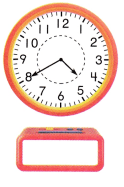Answer: The time on the clock is 5:40

Explanation: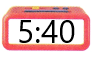The hour hand is between 5 and 6
and the minute hand is on 8 , which makes 40 minutes on the clock ,
for example , if the minute hand is on 6 , makes 30 minutes
but here it is on 8 that means add 10 minutes to the 30 minutes which results in 40 minutes
Making it as 5:40 on the clock

Problem Solving

Craw the clock hands to show the time. Then write the time.

Question 7.
Multi-Step My hour hand points between the 3 and the 4. In 25 minutes it will be the next hour. What time is it?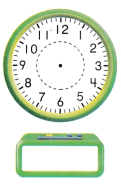Answer: The time on the clock is 3:35

Explanation: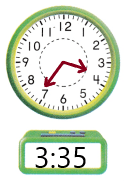The hour hand is between 3 and 4
and the minute hand is on 7 , which makes 35 minutes on the clock ,
for example , if the minute hand is on 6 , makes 30 minutes
but here it is on 7 that means add 5 minutes to the 30 minutes which results in 35 minutes
Making it as 3:35 on the clock

Lesson Check

Question 8.
What time is shown on this clock?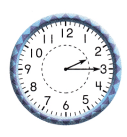(A) 2:15
(B) 2:55
(C) 3:10

Explanation:
The hour hand is between 2 and 3
and the minute hand is on 3, which makes 15 minutes on the clock
That is adding up the 5 minutes three times can make a whole 15 minutes.
Making it as 2:15 on the clock

Question 9.
Kyle looks at the clock. He sees that it is time for story hour at the library. What time is shown on the clock?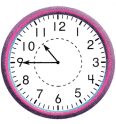(A) 8:50
(B) 10:45
(C) 8:10

Explanation:
The hour hand is between 10 and 11
and the minute hand is on 9 , which makes 45 minutes on the clock ,
for example , if the minute hand is on 6 , makes 30 minutes
but here it is on 9 that means add 15 minutes to the 30 minutes which results in 45 minutes
Making it as 10:45 on the clock

Question 10.
At 3:20, Ray gets on the school bus. Which clock shows that time?Answer: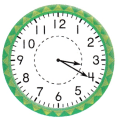Explanation:
The hour hand is between 3 and 4
and the minute hand is on 4, which makes 20 minutes on the clock
That is adding up the 5 minutes to 15 minutes makes 20 minutes
Making it as 3:20 on the clock

Scroll to Top